### Home > PC > Chapter 1 > Lesson 1.1.2 > Problem1-30

1-30.
1. Solve for x in each triangle below. Homework Help ✎

1.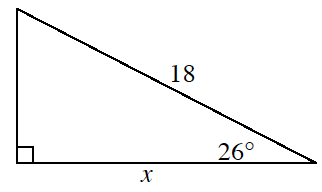2.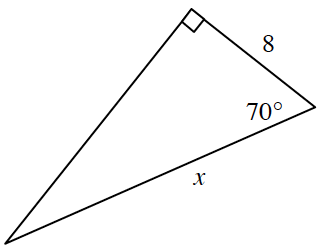3.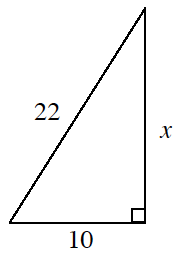4.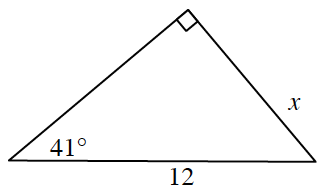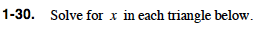$\sin(\theta) = \frac{\text{opposite}}{\text{hypotenuse}}$

$\cos(\theta) = \frac{\text{adjacent}}{\text{hypotenuse}}$

$\tan(\theta) = \frac{\text{opposite}}{\text{adjacent}}$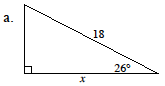Is x the adjacent side or the opposite side in comparison to the 26º angle?

Write an equation involving the trigonometric ratio with the given sides and angle.

$\text{cos(26\degree)} = \frac{x}{18}$

Solve for x. (Use a calculator.)

x ≈ 16.178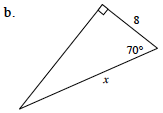Follow the same steps in part (a).

x ≈ 23.390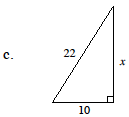Three sides are given, so in this case, use the Pythagorean Theorem.

x ≈ 19.596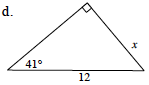Follow the same steps in part (a).

You should have used the sine ratio.
x ≈ 7.873